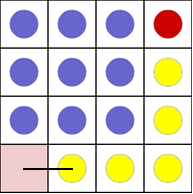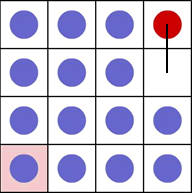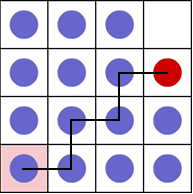#### You may also like### Just Rolling Round

P is a point on the circumference of a circle radius r which rolls, without slipping, inside a circle of radius 2r. What is the locus of P?### Coke Machine

The coke machine in college takes 50 pence pieces. It also takes a certain foreign coin of traditional design...### Just Opposite

A and C are the opposite vertices of a square ABCD, and have coordinates (a,b) and (c,d), respectively. What are the coordinates of the vertices B and D? What is the area of the square?

# Changing Places

##### Age 14 to 16Challenge Level

We had a number of partial solutions, including some from The Mount School in York. The two correct solutions which were sent in from David of the Wreake School and Andrei of Tudor Vianu National College have been used as the basis of what is below. Well done David and Andrei. It looks as if the animation helped to see what was going on and to develop a strategy that could then be represented in algebraic form.

For a 4x4 array, it was possible to complete the change in 21 moves, which the animation said was the minimum.Trying for grids from 2x2 to 5x5, the results are written in the following table:
 Array Moves 2x2 5 3x3 13 4x4 21 5x5 29
It is clear that the difference in the number of moves is always 8.
Now, to predict the necessary number of moves.
Let's imagine the square grid of side n. As 5 is the starting number, and 8 is taken (n-2) times, we obtain:
5 + 8(n-2) = 8n -16 + 5 = 8n- 11
That is for a grid of size n x n the number of moves seems to be from the pattern 8n-1.
However, to prove this general formula,we need to analyse the moves: a) the blue counters from the figure below:The sketch is done for a 4 x 4 array, but it could be generalised for an n x n array.
To make the first space for the red counter we need to create a space below it. This requires you to move the counters (coloured yellow here) along the last row and down the last column.
In an n x n array, there are blue counters on every place of two sides, except the ends, and one in the place of intersection of the two sides.

So, in total there are: 2(n- 2) + 1 = 2n- 4 + 1 = 2n -3 blue counters needing that number of moves.

b) Now the red counter can move one place down:c) there is a series of repeated moves from now on:

There is a pattern which means that the red counter will move every third go, so that the red counter travels around the diagonal of the array.

In the figure below the path of the red counter from the last position, to home is represented.Overall, from this position, the red counter moves n - 2 squares down and n - 1 squares across. So we have:
n - 1 + n - 2 =2n - 3

Each time the red counter moves, two blue counters have to be moved to fill the empty space and create a new one that the red counter can move into. So, we obtain:
3(2n- 3).

Now, adding all the results to calculate the total number of moves:

(2n -3) + 1 + 3(2n -3) = 2n -3 + 1 + 6n -9 = 8n -11

Which matches the prediction.

Andrei also noted that the number of moves is always odd because 8n is always even and 11 is odd. For the 4x4 array, the red counter makes its moves on moves 6, 9, 12, 15, 18 and 21.

For the rectangular case, a similar strategy can be used.

Suppose the rectangle has $m$ rows and $n$ columns, with $m> n$.

First of all, we need $m + n - 3$ moves to get the space to next to the red counter. Then, we need use one move to put the red counter in this space.

We can then move $(n-1)$ squares down and $(n-1)$ across, using three moves per squareas before.

Now we must move down the last $(m-n-1)$ squares. This takes 5 moves for each square, as we do not alternate between horizontal and vertical moves. Adding all this up, the total number of moves needed is $$n + m - 3 + 1 + 2 \times 3(n-1) + 5(n-m-1) = 2n + 6m - 13$$